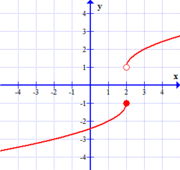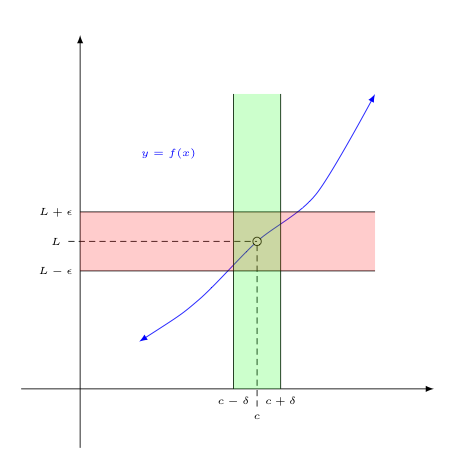## The Epsilon Delta Definition of a Limit

We have said before that we can think of a limit as an "expected" value for a function at some given $x=c$ when the actual behavior there is hidden from us.

In forming that expectation, we assume the graph of the function can be drawn near that point with a single continuous stroke of the pen. This assumption lies at the heart of how we can quickly approximate limiting values for a function given its graph.

However, we can't rigorously define what we mean by a limit in this way. Doing so would be entirely too subjective!

What we need is a clear, precise, and unambiguous definition for a limit that is consistent with the intuitive notion we have developed.

Too often, if you ask someone who has had a brief exposure to Calculus what a limit is, they will tell you (incorrectly) that the limit of a function at some value $c$ is defined as the value that $f(x)$ approaches as $x$ approaches $c$.

After a moment's thought, however, you should realize this would actually be a terrible definition -- one that is not at all consistent with our notion of a limit as an "expected height or value".

Consider the following two functions:

 Graph A$$y=f(x)$$ Graph B$$y=g(x)$$ In graph $A$ above -- if we use this bad definition -- we are forced to accept that $\lim_{x \rightarrow 2} \,\, f(x) = 0$. After all, as $x$ approaches $2$ from either side, the height of the function approaches $0$. Indeed, one could argue that the limiting value must also be 1/2, -1/3, and a multitude of other values too! Clearly there is a problem here. Intuitively, knowing that we have a gap, or jump discontinuity at $x=2$, we should have two different, and conflicting "expectations" for the value of $f(2)$ when the actual behavior there is hidden from us. With no reason to pick one over the other, and hence no single "expected value", this limit should not even exist! In graph $B$ above, on the other hand, shows us the reverse situation -- even when the $x$-values considered are very close to $0$ -- there are always times when $f(x)$ is moving farther away from a height of zero as $x$ approaches zero. Thus, if we use this bad definition, we are forced to conclude that $\lim_{x \rightarrow 0} g(x) \neq 0$. However, given the damped amplitude of the function around $x=0$, our intuition tells us to "expect" $f(0)=0$, so the limit really should exist.

Think carefully about both of the cases above. Do you see why what is being said is true?

So the question now is: "How do we fix what went wrong in the above attempt to better define the notion of limit?"

Notice, in graph $A$, $f(x)$ never gets any closer than one unit away from its (incorrectly) stated limiting height of zero. With our intuitive notion of a limit, we really need to be able to get arbitrarily close to the limiting height.

Also, in graph $B$, the direction $f(x)$ is heading (i.e., either approaching or getting farther away from the limiting height) shouldn't matter near as much as the fact that we can keep $f(x)$ close to the limiting height, provided the $x$-values we consider are sufficiently close to the $c$-value in question.

Putting these two ideas together with the fact that we really don't care what happens to $f(x)$ at $x=c$ gives us a working definition:

 A Better Definition for the Limit of a Function $\lim_{x \rightarrow c} \,\, f(x)=L$ means that we can keep $f(x)$ arbitrarily close to $L$, by keeping $x$ sufficiently close to $c$, with the possible exception of what happens at $x=c$.

This is a good definition, but is it the most useful? How can one, for instance, use this to verify a limit's value? To cite a particular example, how would you show -- using the above definition -- that $\lim_{x \rightarrow 2} \,\, x^2 = 4$ ?

The following definition, while perhaps a bit harder to follow, is completely equivalent to the one above, and -- believe it or not -- is actually easier to work with when trying to verify the value of a limit.

 The Epsilon-Delta Definition for the Limit of a Function $\lim_{x \rightarrow c} \,\, f(x)=L$ means that for any $\epsilon>0$, we can find a $\delta>0$ such that if $0<|x-c|<\delta$, then $|f(x)-L| < \epsilon$.To see the equivalence of the two definitions given, a few comments are in order:

• First, $\epsilon$ provides us with a measure of how "arbitrarily close" to $L$ we wish $f(x)$ to be. In the context of the picture above, $\epsilon$ determines the height of the "red band" about $L$.

Notice that $|f(x)-L|$ is the distance between the height of the function at some $x$ and the height $L$. So, $|f(x)-L| < \epsilon$ tells us that the height of $f(x)$ is staying within $\epsilon$ units of a height of $L$.

In other words, when $|f(x) - L| < \epsilon$ holds, the value of $f(x)$ is being kept in the desired $\epsilon$-band about $L$.

• Second, $\delta$ provides us with a measure of how "sufficiently close" to $c$ we must keep $x$. In the context of the picture above, $\delta$ determines the width of the "green band" about $c$.

Notice that $|x-c|$ is the distance between $x$ and $c$. So, $|x-c|<\delta$ tells us that $x$ is staying within $\delta$ units of $c$.

So $|x-c| < \delta$ tells us that we have chosen an $x$ in the green $\delta$-band.

• We require $\epsilon > 0$ as:

1. distances must never be negative; and

2. allowing one to insist $f(x)$ be zero units away from $L$ when $x$ is sufficiently close to $c$, would force the function to be constant in some small interval around $x=c$, which clearly violates our intuitive notion of what a limit should be.

• We require $\delta > 0$ for similar reasons:

1. $\delta$ also represents a distance, so it can't be negative; and

2. $\delta$ can't be zero, as then the only $x$ value we would be considering would be $c$ itself, which is precisely the value of $x$ we don't care about!

• Finally, we restrict our conclusions to $0 < |x - c|$ to purposefully avoid placing any restrictions on what happens at $x=c$. Remember, in the first definition, what happens there is a "possible exception".